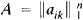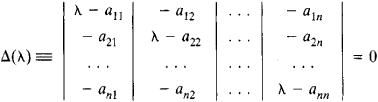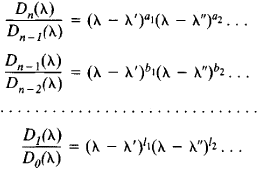Elementary Divisor

Elementary Divisor

The elementary divisors of a square matrixare the polynomials (λ – λ1)p1, (λ – λ2)p2, . . ., (λ – λs)ps obtained from the characteristic equationThe minors of the kth-order determinant Δ(λ), kn, are polynomials in λ. Let Dk (λ), k = 1, 2,. . ., n, be the greatest common divisor of these polynomials, with Dn (λ) ≡ Δ(λ). In the sequence

D0(λ) ≡ 1, D1(λ), D2(λ),...,Dn(λ)

each polynomial is divisible by the preceding one without remainder. Let the corresponding quotients be expressed as a product of linear factors in the field of complex numbers:The polynomials (λ – λ′)a1, (λ – λ″)a2, . . ., (λ – λ′)l1, (λ – λ″)l2, . . . form the complete system of elementary divisors of A (here, powers with zero exponents are not taken into consideration).

The product of all elementary divisors is equal to the characteristic polynomial. The elementary divisors determine the Jordan form of A.

References in periodicals archive ?
We note that obtained results are true for the matrix equation AX = B, where A and B are m-by-n matrices over an elementary divisor domain.
Helmer, "The elementary divisor theorem for certain rings without chain condition," Bulletin (New Series) of the American Mathematical Society, vol.
Kaplansky, "Elementary divisors and modules," Transactions of the American Mathematical Society, vol.
The invariants for two-component handlebody-links are elementary divisors of a matrix whose entries are linking numbers of closed circles corresponding to the basis of one homology group and that of the other homology group.
Then we consider the elementary divisors [d.sub.1]|[absolute value of [d.sub.2]] ...
The elementary divisors of M are 1 | 2 | 4 up to signs.
Therefore we can choose bouquet graphs such that the non-zero entries of the linking matrix of their loops is exactly the elementary divisors of the matrix.
There is a compact formula for the elementary divisors of the Cartan matrix [C.sub.n] [=.sup.t] [D.sub.n][D.sub.n] (10):
We also see that the elementary divisors of [A.sup.(p).sub.n] coincide with
Yamada, Elementary divisors of Cartan matrices for symmetric groups J.

Site: Follow: Share:
Open / Close